# MCAT Physical : Buffers

## Example Questions

### Example Question #43 : Acid Base Chemistry

Which of the following would be most useful as a buffer?

Potassium hydroxide

A solution of carbonic acid and sodium chloride

Water

A solution of sodium chloride and sodium hydroxide

A solution of ammonia and ammonium chloride

A solution of ammonia and ammonium chloride

Explanation:

A buffer must contain either a weak base and its salt or a weak acid and its salt. A mixture of ammonia and ammonium chloride is an example of the first case, since ammonia, NH3, is a weak base and ammonium chloride, NH4Cl, contains its salt.

Though autoionization of water produces small amounts of H3O+ and OH-, each conjugate salts of H2O, they exist in such small amounts as to make any buffering effects negligible.

### Example Question #27 : Acids And Bases

Carbonic anhydrase is an important enzyme that allows CO2 and H2O to be converted into H2CO3. In addition to allowing CO2 to be dissolved into the blood and transported to the lungs for exhalation, the products of the carbonic anhydrase reaction, H2CO3 and a related compound HCO3-, also serve to control the pH of the blood to prevent acidosis or alkalosis. The carbonic anhydrase reaction and acid-base reaction are presented below.

CO2 + H2O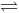H2CO3

H2CO3HCO3- + H+

HCO3- may be considered a(n) __________.

second messenger

buffer compound

enzyme

intermediate

buffer compound

Explanation:

First, we need to determine from both the information presented in the paragraph and the chemical equation what HCO3- is doing. The paragraph tells us “HCO3- also [serves] to control the pH of the blood.” This is the definition of a buffer. A buffer is able to mitigate the addition of acid or base to a solution, or in this case, blood, by being deprotonated or protonated. For example, if the blood becomes too acidic, H+ will recombine with HCO3- to form H2CO3, thus stabilizing the pH. Alternatively, if the pH becomes too basic, H2CO3 will dissociate, releasing H+ into solution.

### Example Question #28 : Acids And Bases

HCN dissociates based on the following reaction: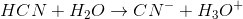The Ka for hydrogen cyanide is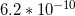.

Two moles of HCN are mixed with one mole of NaCN, resulting in 1L of solution. What is the pH of the solution?

5.1

7.2

4.6

8.9

8.9

Explanation:

Whenever a concentration of the conjugate base is initially present in the solution, we say that the solution has been buffered. NaCN will completely dissolve in solution, meaning that there will be 1M of CN- ions in the solution initially (one mole of NaCN in 1L of soultion equals 1M). With this in mind, the new equilibrium equation for the acid's dissociation is as follows: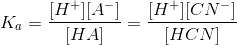The initial concentrations of CN- and HCN are given in the question as 1M and 2M, respectively. The initial concentration of H+ is zero. As HCN begine to dissociate, H+ and CN- will each increase by X amount, while HCN will decrease by X amount.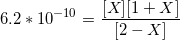Since 1M and 2M are much larger numbers than the value for X, the X values next to these numbers can be omitted from the equation.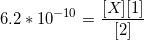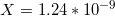By plugging this value into the equation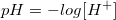, we determine that the pH of the solution is 8.9. (Remember that X is equal to the amount of increase in H+ ions).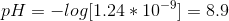This may seem wrong, because HCN is an acid, and we are used to acids resulting in solutions with pH values less than 7; however, HCN is a weak acid, and a large concentration of its conjugate base can result in a basic solution.

### Example Question #44 : Acid Base Chemistry

The pH of a buffered solution is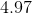. What is the approximate ratio of the concentration of acid to conjugate base if the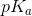of the acid is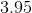?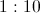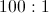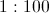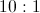Explanation:

In a buffered solution, when the concentrations of acid and conjugate base are equal, we know the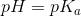.

This is derived from the Henderson-Hasselbalch equation: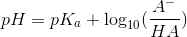.

When concentrations are equal, the log ofis, and.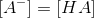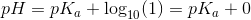In our question, the pH is approximately one unit greater than the.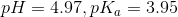Because of this, we know that the log of the two concentrations must be equal to one.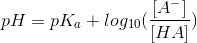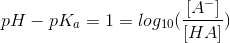The log ofis, and therefore the conjugate base must be ten times greater than the acid; therefore the ratio of acid to base is approximately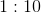.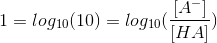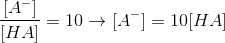We can quickly narrow this question to two possible answers, since the buffered solution is more basic than the, and thus we would expect there to be a greater concentration of base than acid in the solution.

### Example Question #45 : Acid Base Chemistry

Acids and bases can be described in three principal ways. The Arrhenius definition is the most restrictive. It limits acids and bases to species that donate protons and hydroxide ions in solution, respectively. Examples of such acids include HCl and HBr, while KOH and NaOH are examples of bases. When in aqueous solution, these acids proceed to an equilibrium state through a dissociation reaction.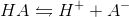All of the bases proceed in a similar fashion.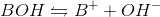The Brønsted-Lowry definition of an acid is a more inclusive approach. All Arrhenius acids and bases are also Brønsted-Lowry acids and bases, but the converse is not true. Brønsted-Lowry acids still reach equilibrium through the same dissociation reaction as Arrhenius acids, but the acid character is defined by different parameters. The Brønsted-Lowry definition considers bases to be hydroxide donors, like the Arrhenius definition, but also includes conjugate bases such as the A- in the above reaction. In the reverse reaction, A- accepts the proton to regenerate HA. The Brønsted-Lowry definition thus defines bases as proton acceptors, and acids as proton donors.

A scientist is making a buffer to maintain the pH of a solution at about 6. Which of the following describes the best composition of the solution?

A larger amount of acid , with some conjugate base, where the acid has a pKa of 4.9.

A larger amount of acid , with some conjugate base, where the acid has a pKa of 5.9.

About equal amounts of acid and conjugate base, where the acid has a pKa of 5.9.

A larger amount of acid , with some conjugate base, where the acid has a pKa of 6.9.

About equal amounts of acid and conjugate base, where the acid has a pKa of 6.9.

About equal amounts of acid and conjugate base, where the acid has a pKa of 5.9.

Explanation:

A buffer is best made of equal and copious amounts of an acid and its conjugate base. The acid must have a pKa as close as possible to the pH desired. For this question, the desired pH is 6. The best option will have a pKa of about 5.9.

Adding larger amounts of the acid than the base will skew the equilibrium in favor of the acid, increasing the overall proton concentration, and limiting the buffer ability to absorb more proton addition without changing pH significantly.

### Example Question #1 : Buffers

Which of the following would be a good buffer solution?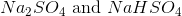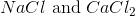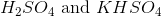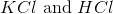Explanation:

A good buffer solution consists of a weak acid with its conjugate base, or a weak base and its conjugate acid. A strong acid or base will never be a good buffer. We will go through each answer choice in detail.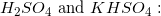Sulfuric acid is a strong acid and cannot be used in a buffer.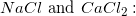Neither sodium chloride, nor calcium chloride constitute a weak acid or weak base. Both of these are simple salts and will not be able to create a buffer solution.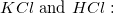Hydrochloric acid is a strong acid and cannot be used in a buffer.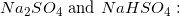In solution, these salts will produce sulfate ions and hydrogen sulfate ions. Hydrogen sulfate is a weak acid and sulfate is its conjugate base. These ions will form a buffer solution.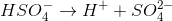### Example Question #1 : Help With Buffers

Which of the following pairs cannot constitute a buffer system?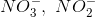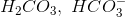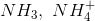Explanation:

A buffer system is composed of one of the following scenarios:

1. A weak base with its conjugate acid.

2. A weak acid with its conjugate base.

The only option that does not qualify is the nitrate, nitrite pair. A difference of one oxygen does not make a buffer system.